# 如何在 COMSOL Multiphysics® 中模拟不同类型的阻尼

2019年 3月 15日

### 如何在有限元分析中添加阻尼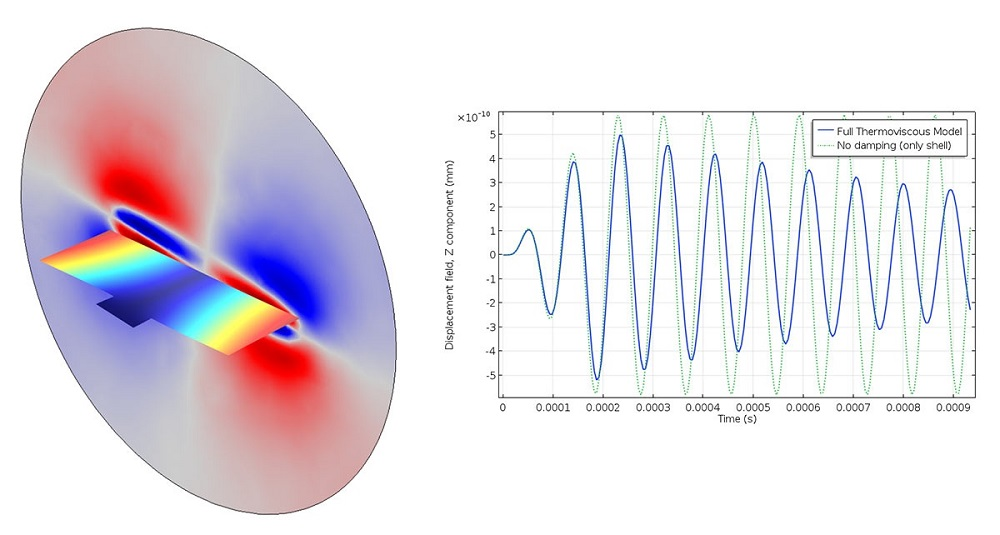#### 特征频率分析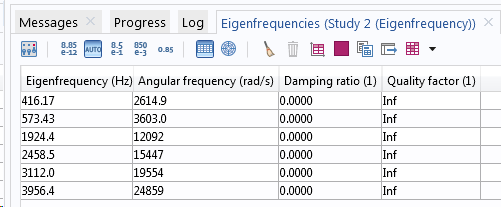### 阻尼的数值模型

#### 有限元公式

\mathbf M \ddot {\mathbf u} + \mathbf C \dot {\mathbf u} + \mathbf K {\mathbf u} = \mathbf f(t)

\left ( -\omega^2 \mathbf M + i \omega \mathbf C + \mathbf K \right ) \tilde { \mathbf u} = \tilde {\mathbf f}

#### 阻尼损耗因子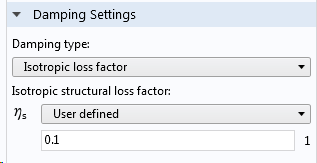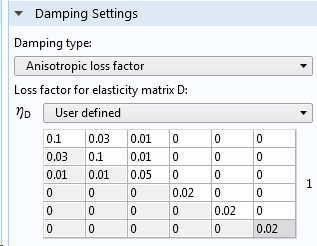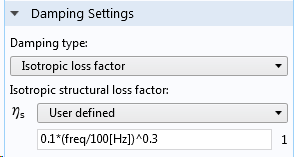\mathbf C = \dfrac {\eta \mathbf K}
{\omega}

\left ( -\omega^2 \mathbf M + (1+ i \eta) \mathbf K \right ) \tilde { \mathbf u} = \tilde {\mathbf f}

#### 黏滞阻尼

COMSOL Multiphysics 中固体力学 接口中的黏滞阻尼使用两个常数：

• 本体黏度，\eta_b
• 剪切黏度，\eta_v

\boldsymbol {\sigma}_v = \eta_b \dot \epsilon_v \mathbf I +\eta_v \dot {\boldsymbol \epsilon}_d#### 瑞利阻尼

\mathbf C = \alpha \mathbf M + \beta \mathbf K

\eta_b =\beta K
\eta_v =\beta G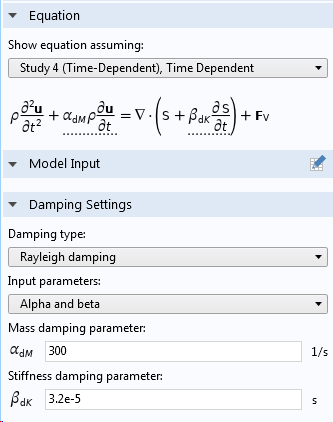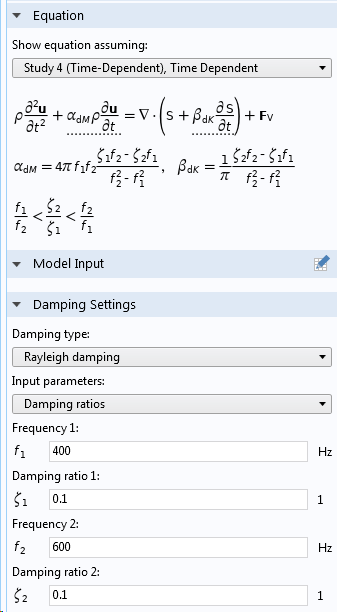#### 损耗材料模型#### 热弹性阻尼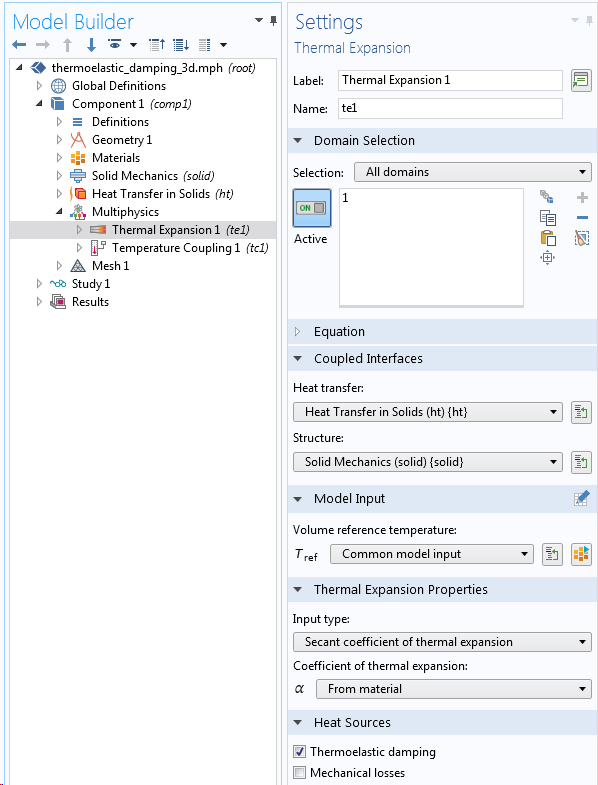Q=-T \dot{\boldsymbol \sigma}: \boldsymbol \alpha

#### 模态阻尼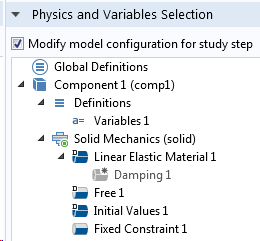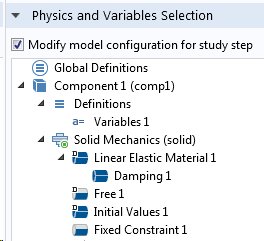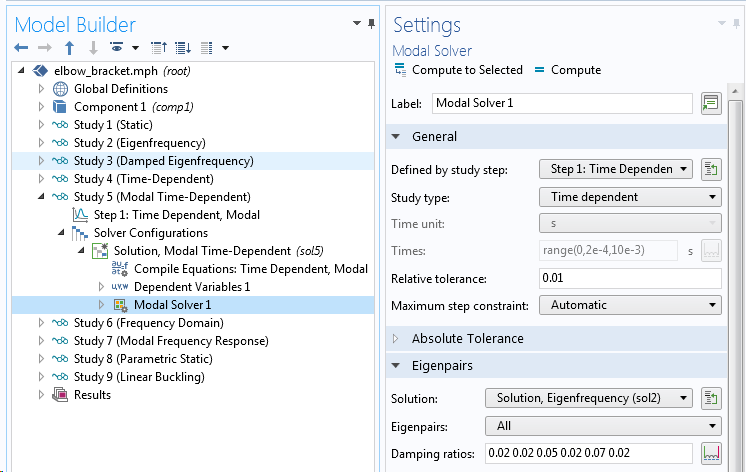#### 无限边界条件

PML 是使用计算域外部的少量单元层模拟的。• 可用于时域分析
• 由于这是一个边界条件，因此不需要在计算域之外对额外的域进行网格剖分#### 使用其他功能添加阻尼

• 弹簧基础 功能
• 弹性薄层 功能
• 弹簧阻尼器 功能
• 多体动力学 接口中的关节和齿轮
• 集总机械系统 接口中的阻尼器阻抗 功能
• 转子动力学多体动力学 接口中的轴承
• 速度依赖的任何载荷
• 复数值的材料数据

### 下一步

#### 评论 (4)

##### 留言##### JC Shark
2022-11-01

Where can I get this example?##### Qihang Lin
2022-11-02 COMSOL 员工##### 松祥 纪
2023-09-20##### Liwen Yang
2023-09-25 COMSOL 员工